# Introduction to Transformers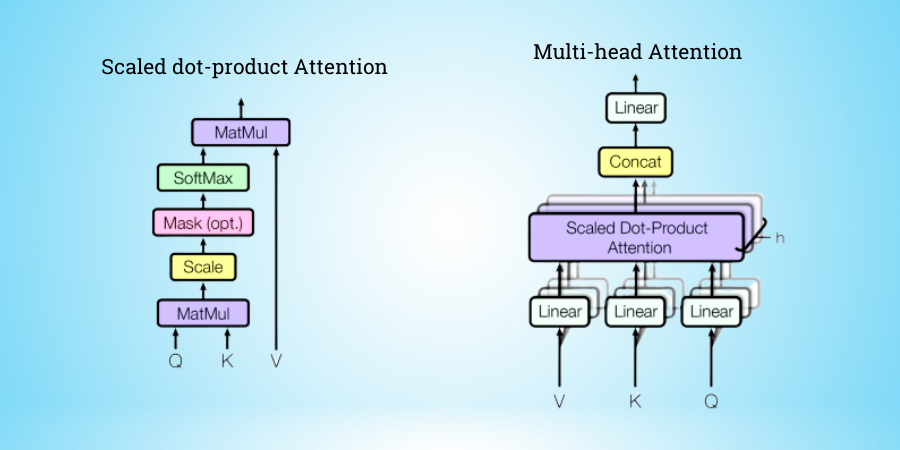S.No Lesson Title
1
2
3
4
5
6

### Introduction

Convolutional neural networks have been used in computer vision since the 1980s but the main breakthrough came when Alexnet won the Imagenet challenge in 2012. The success of Alexnet was due to two main reasons:

1. Availability of Imagenet Dataset
2. Use of commoditized GPU hardware

Since then, CNNs have become the go-to model choice for almost all vision tasks. The main advantage of using CNNs is that they avoid the need for hand designed features and instead make an end to end pipeline. However, the architecture is made specifically for images. Looking at the current advancements in computer vision, you might ask if we can make CNNs more domain agnostic and computationally efficient. This leads to another type of neural network known as Recurrent Neural Networks (RNNs). Here, I would recommend you to read about RNNs and related architectures: Long short term memory networks(LSTMs) and Gated recurrent units(GRUs).

RNNs were at the top of the leading approaches for natural language processing tasks like language modelling, machine translation, question answering etc. but since the release of transformers, it has become the go-to choice rather than RNNs.

### Limitations of RNNs

I assume you are familiar with RNNs, LSTMs and GRUs. In particular, LSTMs and GRUs were popular RNNs to encode rich semantics from words in a text. They work in sequential manner processing one token at a time step. We call words in a sentence as tokens. Along with the processing, they also maintain a memory of all the previous tokens that the model has already processed. This allowed our model to solve the vanishing and exploding gradient problems and also add the semantics of previously processed tokens in the further words that require them to solve the problem of long dependencies. But storing memory for future tokens and processing tokens sequentially is not very suitable when we really have very long dependencies.

The sequential nature of the model also makes it difficult to scale and parallelize efficiently and this is the major limitation of RNNs. In the next topic, we will cover how the limitation of RNNs can be solved.

### Need for Transformers

Transformers arrived in the year 2017 as a simple and scalable way to obtain State-of-the-art results in language translation. Since then, they have become the SOTA for most of the NLP related tasks such as image captioning, language modelling etc. The biggest limitation of RNNs/LSTMs/GRUs is the biggest benefit that comes by using transformers i.e. transformers do not process one token at a time. This simply means transformers are not sequential and they can be parallelized. Also, transformers use a self attention mechanism which directly models relationships between all words in the sentence. I would recommend you to read about self attention. Here is a small illustration -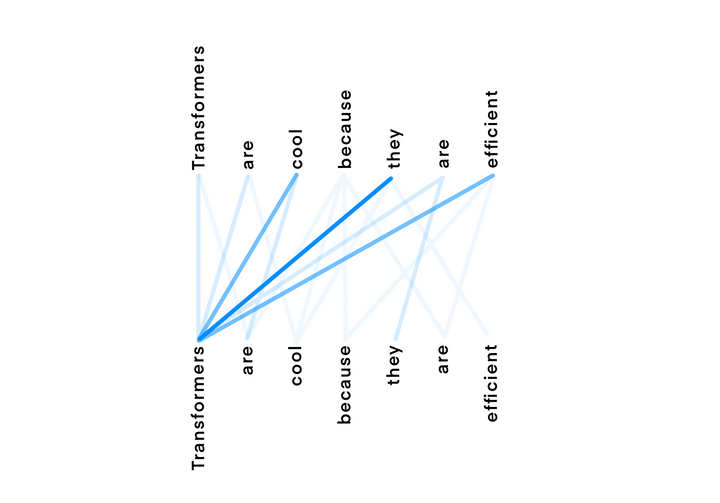In the above illustration, the words on the top are being processed and the words in the bottom are used as context. So the word 'they' in the top row is using 'transformer' as its context. Now, let us understand the architecture of transformers.

### Architecture of Transformers

The transformer was proposed in the paper 'Attention is all you need'. Harvard's NLP group has created a very good guide explaining the paper along with its implementation. Here is the link and I would recommend you to go through this amazing explanation by Harvard.

http://nlp.seas.harvard.edu/2018/04/03/attention.html

Let us now try to understand the architecture. Consider the below image as Fig1, showing a high level architecture of the transformer. As we move through this article, we will unpack things one by one.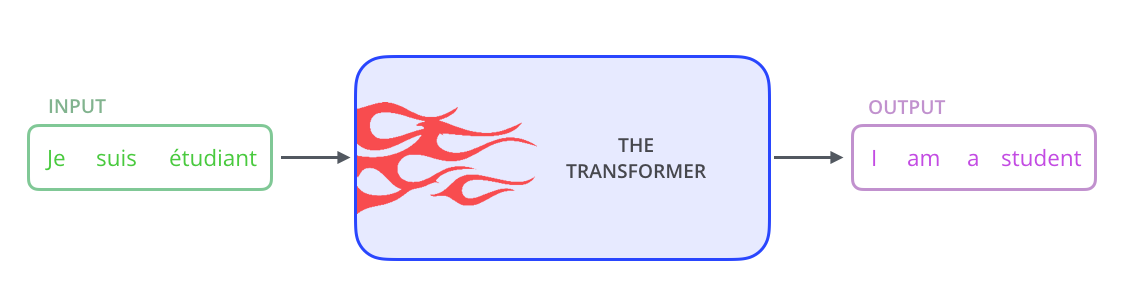In the above image, consider 'THE TRANSFORMER' as a black box which is translating a given statement in one language to another language. If we now look inside the black box, we will see Encoders and Decoders. Below is the illustration.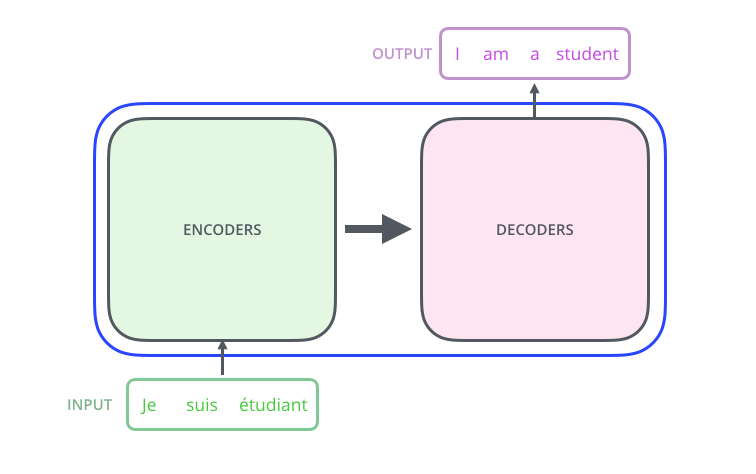If we further look into the encoders and decoders, we will find a stack of them. Look below for the illustration.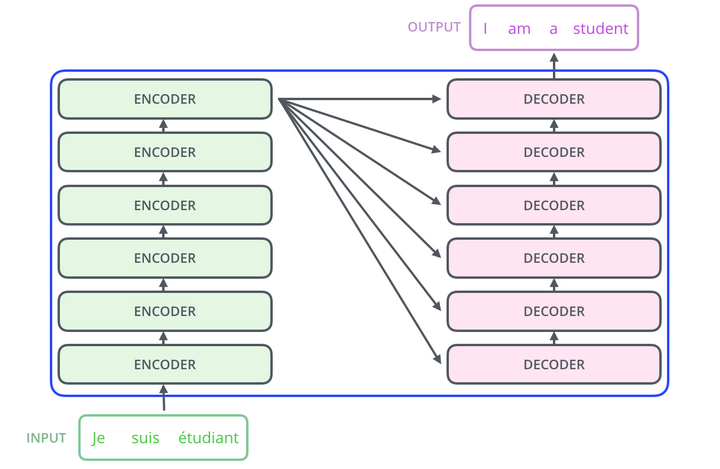In the above picture, the number of encoders and decoders are six but it is not compulsory. You can play around with this number as per your sequential model . All Encoders are identical but they have different weights and 2 sublayers which are self attention layer and feed forward neural network layer. The inputs of a transformer are first fed to the self-attention layer and the output of the self attention layer is passed to a neural network. We will discuss self attention in detail as we move through the article. Similarly, all decoders are also identical and have 3 sublayers. Below image shows 2 layers of an encoder and 3 layers of the decoder which are : self attention layer, Encoder-Decoder attention and NN layer.Combining all this information into one model will give us the Transformer architecture. Here is the illustration.

6transformers-6.png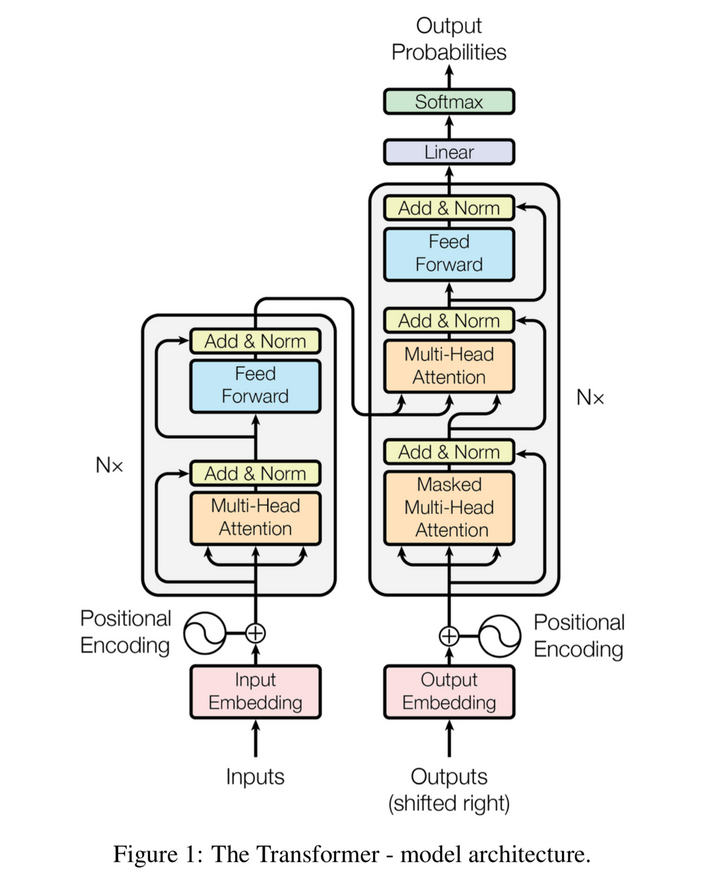Now, let us understand encoders in detail. As I mentioned earlier, each encoder has one attention layer and a neural network layer. The first attention layer takes word embeddings of our input as the input vector while the other attention layers in the subsequent encoders have the output of the neural network as their input.

So, our first task is to generate word embeddings. You are already familiar with methods like t-SNE which can help to generate word embeddings. Please read about t-SNE if you are not familiar.

Let us take the same input which I sent to the black box in the very first image i.e. Fig1. In the below diagram, x1,x2 and x3 are the word embeddings of each word in our input sentence and these embeddings are sent to the self attention layer in the encoder individually.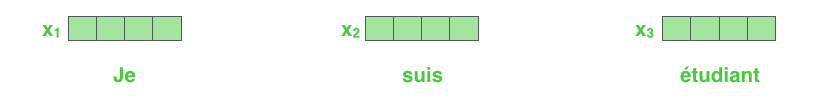Here, we can see the biggest benefit of transformers. These word embeddings have their own path through the self attention layer and feed forward layer. However, the attention mechanism in the self attention layers make them dependent. The feed forward neural network does not have such dependencies and therefore we can execute these paths in parallel. Here is the illustration.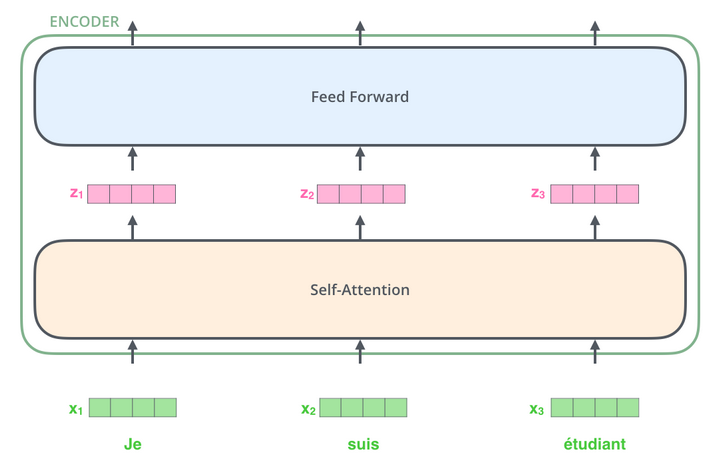The output of the feed forward layer is sent as input to the next encoder. Self attention is all you need! Let us discuss the self attention layer. Consider the following statement -

"The animal didn't cross the streets because it was too tired"

In this sentence, could you tell me the word 'it' refers to ?

For a human, It is quite easy to identify that the word 'it' refers to 'animal'. But it is difficult for an algorithm. So,here we need an attention mechanism. When the algorithm is processing the word 'it', self attention helps it to associate this word to 'animal'. Similarly, as the algorithm processes each word, self attention allows this algorithm to find the similarity between other words and helps to associate.

So the first thing is the input. In this case, our word embeddings will be our input. Now, with this input, we need to create three matrices namely, Query, Key and Value matrix.

You can understand it by an example. Suppose you search something on youtube. That is your query vector. Youtube has a large database which has keys and values. The underlying algorithm tries to find the similarity between your query and each key in the database. This similarity can be calculated in many ways, The simplest approach is to take dot product or Scaled Dot product. The similarity will give you a scalar which is the weight. Then you apply softmax on these weights and multiply this probability with the values and sum them up. The output value gives you the closest key to your query This actually does not happen on Youtube, I have just taken an example to help you understand.

So to generate our Query, Key and Value Matrices, we multiply our input embedding with query, key and value weight matrices. These weights are learned in the training.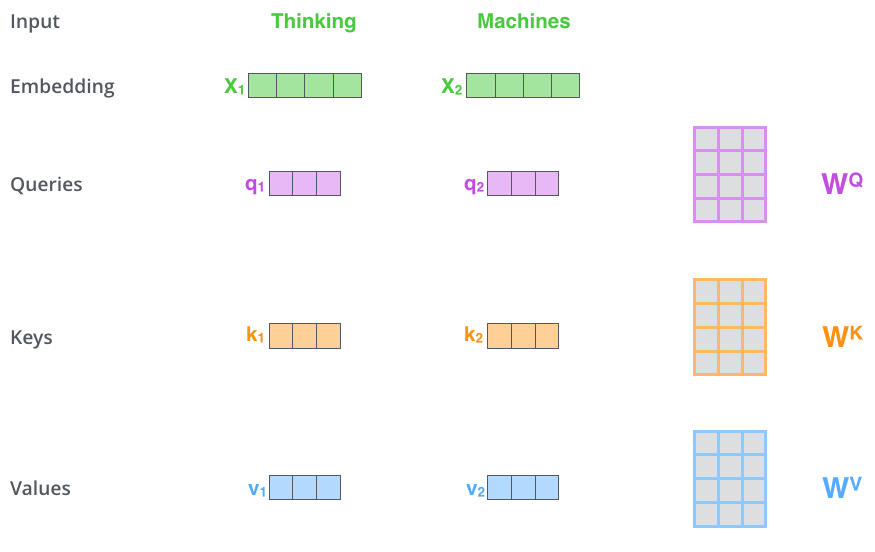In this above figure, q1,q2 are obtained by multiplying x1,x2 to the WQ weight matrix. Similarly, we obtain keys and values.

Next step in the process is to calculate the score for each word to every other word. In the above example, we have only taken two words.

Before discussing further, I would like to add one point for the word embeddings. Their dimension is basically equal to the number of words of the largest sentence in the training data. Continuing on calculating score, generally we take a scaled dot product between the key and query matrix.

In the above figure, you can see the score which is further divided by 8. We call this a scaled dot product. Without the scaling factor, it would simply be a dot product which is also one of the methods to calculate score.After we have found the similarity between keys and queries, we apply a softmax to calculate the probability. Next, we multiply this probability with the value matrix and add the value matrices. The softmax will give the highest probability to the word itself but in many cases, it is helpful to look at other word probabilities also. Like in the case of 'it in the example discussed earlier'. Then, we pass the output of the self attention layer to the neural network.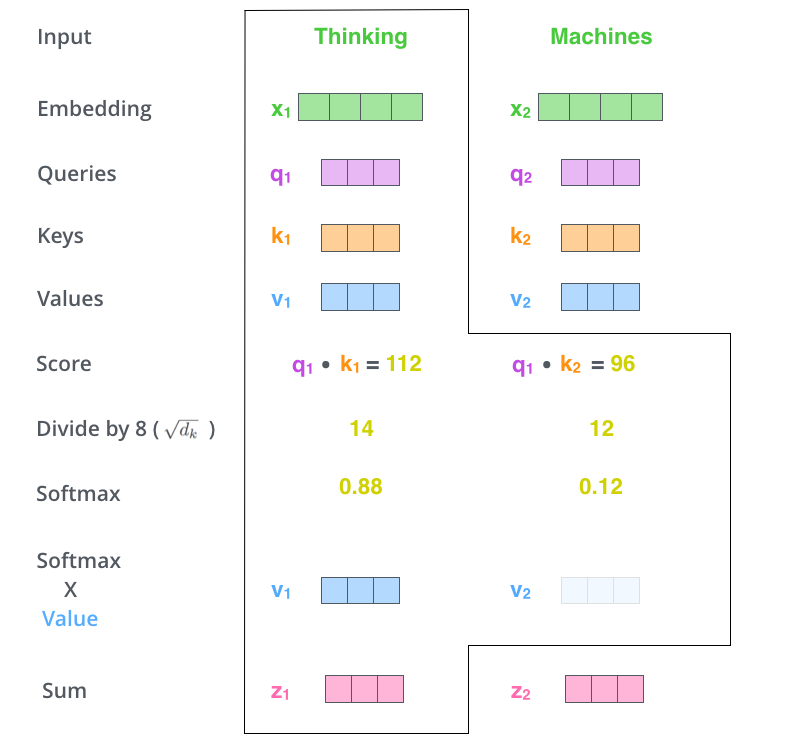There is also a multi headed attention mechanism, In the self attention mechanism, the output of self attention layer z's can be dominated by the word itself. So to avoid that problem, we can use a multi-headed attention mechanism. The picture below will help you to understand it better.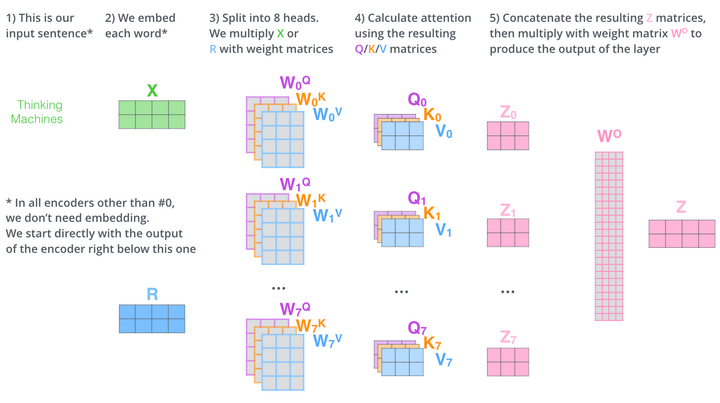As you can see, we have 8 keys, query and value matrices which will give us 8 queries,keys and values and 8 Z matrices output. We also learn (Wo) as part of the training process and compute our final Z. This way we can eliminate the dominance of individual words.

That's all for the self attention layer.

Things that are left in the encoder structure are position encoding, layer normalization and residual connection. Position encodings add a position vector to the word embeddings which are otherwise random. You can read more about positional encoding in the research paper Attention is all I Need. Layer Normalization is similar to the batch normalization except that it computes the mean and variance over the data in the same layer. So all the matrices are normalized to have 0 mean and variance 1. This allows for faster generalization of our algorithm.

In the image given below, you can see the input word embeddings concatenated with positional encodings, passed to the self attention layer whose output is added with a residual connection followed by layer normalization and passed to the neural network. At the end, there is again a layer normalization and the output is given as input to the next encoder on the stack.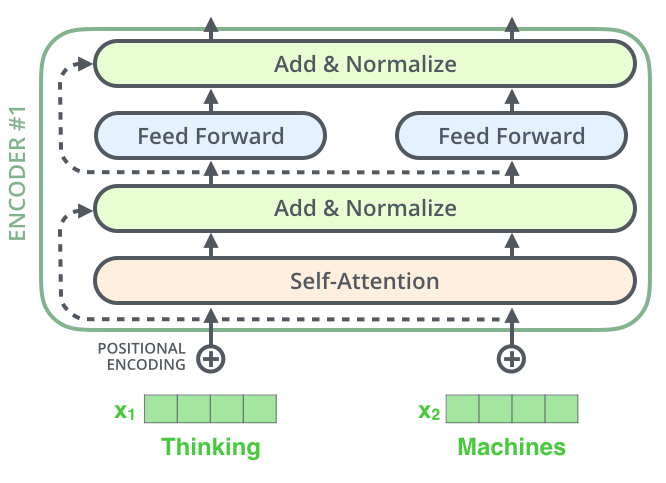The Decoder is also similar to the encoder. The output of the encoder is converted into K and V attention vectors. These are used by encoder - decoder attention in the decoder architecture which helps decoder to focus on appropriate input. The output is the probability of the next word in the sentence. That word embedding is then sent to the attention layer of the decoder after positional encoding.

The attention layer in the decoder is slightly different from the self attention layer of the encoder. Here we do not take the unseen output in our probability calculation. So we add negative infinity before the softmax step to mask future positions. Now, let us do a mini project on transformers.

### Training

You can also visit the link below for understanding the training process of transformers.

### Conclusion

That's all from my side. It is a long article but it will make you well versed with the transformers. Transformers are used in most of the NLP tasks today. You can refer to the references given below.